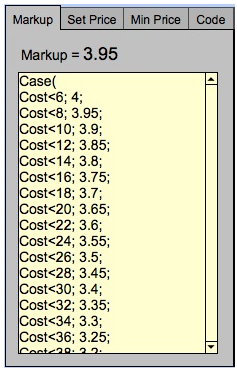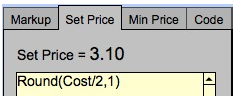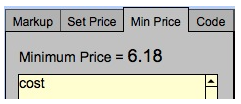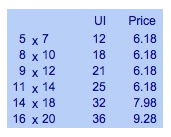### Theory

This is how the retail price of a frame is derived:

the wholesale cost of the matboard x markup + set price.

The default formula is applied to all matboard regardless of the board size, e.g. 32×40, 40×60.

To understand this default formula, you will need to look at the markup and set price:

 Wholesale Cost Less Than Markup Cost <6 4 Cost <8 3.95 Cost <10 3.9 Cost <12 3.85

#### Markup Calculation

The markup is determined by the wholesale cost and is setup on a sliding scale. As the cost of the matboard increases, the markup decreases. The wholesale cost level of matboard starts at \$6.00 and increases by \$2.00 all the way up to \$44.00. The lowest markup, for all matboard costing more than \$44.00 is a 3 times markup. The markup increases in small increments of .05 per level. (When markups increase by large increments, is it possible that you will pay more for the mat and receive less profit on it if the markup changes by more than .05.)#### Set Price Calculation

The Set Price is an amount which is automatically added to the retail price of the matboard, regardless of the size being sold. The FrameReady default calculates half of the wholesale cost of the matboard, rounded up to the nearest dollar, e.g. if the wholesale cost is \$10.83, the set price will be \$5.40. The formula allows the set price to be calculated so that when the price of the mat increases the set price will also increase.

The formula for this calculation is:
Round( Cost / 2; 1 )

By using a calculation instead of just a number, the frame shop owner can be assured that at least half the cost of the matboard will be covered, regardless of the size. And the set price will always increase whenever a vendor price update is performed.You may also chose to apply a set price based on Supplier to cover shipping costs.

#### Minimum Price Calculation

The Min Price field is used to set a minimum dollar amount for the retail price of the matboard. The retail price will never fall below the amount in the field. By entering the word “Cost“, the amount is based on the wholesale cost of the mat. In the example below, the cost of the mat is \$6.18.The retail price of the mat will remain the same until it reaches the point where the retail price surpasses \$6.18. At that point, the price will increase as per the calculations of the markup and set price.The is not used in the FrameReady default pricing of matboard.